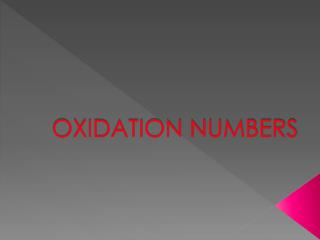DownloadDownload PresentationOXIDATION NUMBERS

# OXIDATION NUMBERS

Télécharger la présentation## OXIDATION NUMBERS

- - - - - - - - - - - - - - - - - - - - - - - - - - - E N D - - - - - - - - - - - - - - - - - - - - - - - - - - -
##### Presentation Transcript

1. OXIDATION NUMBERS

2. What is an oxidation number? • An oxidation number is the charge that an atom in a compound would have if the electron pairs in the bond belonged solely to the more electronegative atom.

3. General Guidelines • The oxidation # of an atom in its pure form, such as Na or Fe, or in a molecule of that element only, such as O2 or P4, is ZERO.

4. The oxidation # of a monatomic ion is the charge on the ion. ex. Ca2+ is +2

5. a) O = -2 except in H2O2 (-1) b) H = +1 except in metal hydride, ex. LiH (-1) c) Group IA = +1 d) Group IIA = +2 e) Halides = -1

6. Use charges on polyatomic ion as indicator, examples: SO42- , NO3- The algebraic sum of the positive & negative oxidation #’s of the atoms in a polyatomic ion is equal to the charge on the ion.

7. SO42- example:

8. The sum of the positive & negative oxidation #’s in a neutral compound is zero. example: K2Cr2O7

9. Examples • What is the single oxidation number of manganese (Mn) in: • MnO2 • Mn2O7 • MnO42- • KMnO4

10. Determine the single oxidation # of the underlined atom in each of the following species: • CrO42- • P4O10 • Fe3O4 • S2O32-

11. Practice Sheet